Activation Energy for the Decomposition of HI Problems

Chemical Kinetics/Reaction Rate

Multiple Choice

1. Select the rate law that corresponds to the data shown for the following reaction:

2 A + 3 B 2 C

Experiment Initial A InitialB Initial Rate

1 0.15 0.12 0.10

2 0.15 0.24 0.20

3 0.45 0.24 1.80

a) R=kA3B b) R=kA3 c) R=kA2B2 d) R=kA2B

1. Consider the following reaction:

A 2 C

The average rate of appearance of C is given by WC/Wt. How is the average rate of

appearance of C related to the average rate of disappearance of A?

a) – 2WA/Wt b) WA/Wt c) – WA/Wt d) – WA/2Wt

1. Which one of the following graphs shows the correct relationship between concentration and

time for a second order reaction?

1. c)

logA 1/A

time time

1. d)

A A

time time

1. Which of the following will lower the activation energy for a reaction?
2. increasing the concentration of reactants
3. raising the temperature of the reaction
4. adding a suitable catalyst
5. all of the above
6. Consider the following reaction and its associated rate law:

A + B C R = k A2

Which of the following will not increase the rate of the reaction?

1. increasing the concentration of reactant A
2. increasing the concentration of reactant B
3. increasing the temperature of the reaction
4. adding a suitable catalyst
5. As the temperature of a reaction is increased, the rate of the reaction increases because the
6. reactant molecules collide less frequently
7. reactant molecules collide with greater energy
8. activation energy is lowered
9. reactant molecules collide less frequently and with greater energy
10. Which line in the reaction profile corresponds to the activation energy for the forward

reaction?

Energy

Extent of Reaction

a) w b) x c) y d) z

1. In any multi-step reaction mechanism, the rate of the overall reaction is determined by the

rate of the ___________ step in the mechanism.

a) first b) last c) slowest d) fastest

1. Consider the following hypothetical chemical reaction:

2 A + 2 B C

The mechanism for this reaction is

1) A + B D (slow)

2) D + B E (fast)

3) A + E C (fast)

Which of the following is the correct rate law for this reaction?

a) R=kAB b) R=kAE c) R=kA2B2 d) R=kDB

1. In the figure below, the quantity x is which of the five choices?

Potential

Energy

Reaction Coordinate

a) E b) Ea (forward) c) Ea (reverse) d) the collision energy

e) the steric factor

1. For a simple two-step elementary reaction:

Step 1 Step 2

Reactants Intermediates Products

the potential energy profile is given below. Which one of the following statements applies?

Potential

Energy

Reaction Coordinate

1. The intermediates accumulate in measurable quantities, and the reaction is easily resolved into two steps
2. The reactants quickly form intermediates that slowly form products.
3. The presence of intermediates is difficult to determine kinetically although the two-step reaction is not ultra-fast.
4. The intermediates are the final products.
5. None of the above.
6. Which substance in the reaction below either appears or disappears the fastest?

4 NH3 + 7 O2 4 NO2 + 6 H2O

a) NH3 b) O2 c) NO2 d) H2O

1. Which one of the following rate laws is for a reaction that is overall third order?
2. rate = kNO3O2 c) rate = kNH33O23

OsO23

b) rate = kC6H82H22 d) rate = kH2O23

H2

1. Calculate the rate constant of a first order process that has a half-life of 225 s.

a) 0.693 s-1 b) 3.08 x 10-3 s-1 c) 1.25 s-1

1. What is the intermediate in the following mechanism?

A + B C + D

B + D X

a) A b) B c) C d) D e) X

1. For the elementary reaction NO3 + CO NO2 + CO2
2. the molecularity is 2 and rate = kNO3CO
3. the molecularity is 4 and rate = kNO3CONO2CO2
4. the molecularity is 2 and rate = kNO2CO2
5. the molecularity is 2 and rate = kNO3CO/NO2CO2
6. the molecularity is 4 and rate = kNO2CO2/NO3CO
7. Given the following mechanism, which species below may be classified as intermediates in the formation of XO2 from X and O2?

X + YO2 XO + YO

XO + YO XO2 + YO

YO + O2 YO + O

YO + O YO2

a) X only b) YO only c) both X & YO2 d) both XO & YO

e) both YO2 & O2

1. Given the following mechanism, which of the species below is a catalyst in the formation of

XO2 from X and O2?

X + YO2 XO + YO

XO + YO2 XO2 + YO

YO + O2 YO2 + O

YO O YO2

a) X b) O2 c) YO2 d) XO e) XO2

1. Chlorine atoms photochemically derived from Freons help destroy stratospheric ozone in the

two-step mechanism below. We classify the respective species Cl and ClO for the overall

balanced reaction as

Cl + O3 ClO + O2

ClO + O Cl + O2

a) both catalysts d) intermediate and catalyst

b) both intermediates e) both activated complexes

1. catalyst and intermediate

Problems (Show all work and include units)

1. Use the data table below to calculate the average rate of the reaction between 10 s and 30 s.

A + B C

Time (seconds) A mol/L

1. 0.124
2. 0.110
3. 0.088
4. 0.073
5. 0.054
6. Assume that the reaction described below is first order in reactants A and B. Calculate the

value of the reaction rate constant using data for experiment 1.

A + 3 B 2 C

Experiment Initial A Initial B Initial Rate

1 0.15 0.12 0.10

2 0.15 0.24 0.20

3 0.45 0.24 1.80

1. The reaction rate constant for a particular second order reaction is 0.47 L/mol s. If the

initial concentration of reactant is 0.25 mol/L, how many seconds will it take for the

concentration to decrease to 0.13 mol/L.

4. The activation energy for the reaction 2 NO2 (g) 2 NO (g) + O2 (g) is 114 kJ/mol. If

k = 0.75 M-1 s-1 at 600.0 0C, what is the value of k at 500.0 0C?

1. The mechanism,

k1

Step1 NO2 + O3 NO3 + O2 (slow)

k2

Step 2 NO3 + NO2 N2O5 (fast)

Has been proposed for a reaction.

1. Write the equation for the overall reaction.
2. Write the rate laws for steps 1 and 2.
3. Write the rate law predicted by the mechanism for the overall reaction.
4. Which species is an intermediate?
5. Determine the molecularity of step 1.

6. The reaction A B + C is carried out at a particular temperature. The observed data is

recorded below. Graph the data to determine the order of the reaction, write the rate law for

the reaction, and determine the value of k at this temperature.

Time (min) A mol/L

0.00 2.000

2.00 1.107

4.00 0.612

6.00 0.338

8.00 0.187

1. 0.103
1. For a certain reaction, the rate constant doubles when the temperature increases from 15.00C

To 25.00C. Calculate: a) the activation energy

b) the rate constant at 100.00C, taking k at 25.00C to be

1.2 x 10-2L/mol s

CHAPTER 13- Practice Exercise Dr. PAHLAVAN

# Chemical Kinetics

Problem #1. Consider reaction; 2N2O (g) 2N2 (g) + O2 (g)

(a) Write three different expressions which might be used to describe rates of reaction.

(b) Relate the three rates to each other and explain the relative rate.

Problem #2. Consider the reaction; 2NO (g) + 2H2 (g) N2 (g) + 2H2O (g)

(a) Write the rate law expression for reaction.

(b) If rate law is = K[NO]2[H2],

I) determine the overall order and solve equation for rate constant.

II) Write unit of K if the unit of concentration is M or atm.

(c) How does the initial rate change if the initial concentration of NO(g) is doubled and that of

H2 (g) remains constant?

(d) How does the initial rate change if the initial concentration of H2 (g) is doubled and that of NO(g) remains constant?

Problem #3. Use the table of data shown below to calculate the average rate of the reaction ; 2NO + Br2 2NOBr

(a) between 10 sec and 30 sec (b) after 25 sec

Time (seconds): 0 10 20 30 40

[NO] mol/L : 0.124 0.110 0.088 0.073 0.054

Problem #4. The half-life of a first order reaction is 6.00×102 s. What is the rate constant for the reaction?

Problem #5. The rate constant for the first-order decomposition of ; 2N2O5 (g) 4NO2 (g) + O2 (g)

at 25 oC is 6.32 x104 s1.

1. What is the concentration of N2O5 remaining after 2.00 hrs, if initial concentration of N2O5 was

0.500M?

(b) How long will it take (time required) for 90% of the N2O5 to decompose?

(c) How long will it take for 90% to remain? (d) How long will it take for 50% to remain?

Problem #6. The following data were collected in three different experiments for the reaction;   A + B C

EXP # [A]0, M [B]0, M initial rate, Ms-1

1 0.20 1.0 5.0×10-2

2 0.20 2.0 2.0×10-1

3 0.40 2.0 4.0×10-1

(a) Determine the rate law for the reaction as a function of the concentrations of A and B.

(b) What is the overall order of the reaction? (c) Determine the rate constant (K) value.

(d) Determine the rate if [A]0 = 0.30 M and [B]0 = 0.10 M.

Problem #7. Given the following kinetic data for the reaction ; 2A + B + C 2D

[A]0, M [B]0, M [C]0, M initial rate, Ms1

0.1 0.1 0.1 1.0

0.2 0.1 0.1 4.0

0.2 0.2 0.1 32.0

0.2 0.1 0.2 8.0

Determine the rate law as a function of concentration of A, B, and C, and determine the overall order of the reaction.

Problem # 8. The differential rate law for the association of iodine atoms to molecule iodine, 2I (g) I2 (g)

is given rate = k[I]2 , where k = 7.0 x 109

a. What are the proper units for k? b. If [I]0 = 0.40 M, calculate[I] at t=2.5×107 sec.

1. If [I]0 = 0.40 M, calculate t1/2.

d. If [I]0 = 0.80 M, how much time would it take for 75% of the iodine atoms to react?

Problem # 9. a) Consider the first order reaction 2N2O5(g) 2N2O4 (g) + O2 (g) . The frequency factor A is 8.7×1012 s-1 and the activation energy is 63 kJ /mol. What is the rate constant for reaction at 75 oC?

b) Consider the decomposition of N2O5 from the following data:

N2O4 (g) 2NO2 , Ea = 108 kJ/mole

at T = 35o C k = 6.60×105 s-1. Calculate temperature when k = 2.40×103 s-1

Problem #10. Calculate the activation energy for the decomposition reaction of NO2 (g): 2NO2 (g) 2NO (g) + O2 (g)

given that the rate constant at 300 oC is 5.7 x 101 M1s1 and at 230 oC it is 2.7 x 102 M1s1.

Problem # 11. An alteration in the structure of a certain virus follows first-order kinetics with an activation

energy of 587 kJ/mol. The half-life of the reaction at 29.6 0C is 1.62×104s (1yr = 3.154 x 107s).

What are the rate constant at 29.6 oC and the half-life at 32.0 oC for the alteration of structure

of the virus?

Problem # 12. Consider the following elementary reactions (process)

I) CO (g) + Cl2 (g) COCl2 (g) II) HClI (g) HCl (g) + I (g)

What is the molecularity of each reaction and write the rate law expression for each?

Problem # 13. Given the following mechanism for a reaction.

I) Cl2 (g) 2Cl (g) ( slow)

II) 2NO (g) + 2Cl (g) N2 (g) + 2ClO (g) (fast)

III) 2ClO (g) Cl2 (g) + O2 (g) (fast)

(a) Identify intermediate and catalyst (if any). (b) What is the molecularity of step(III)?

(c) Which step determines the reaction rate and write rate law for that step.

(d) Write the overall reaction equation and write rate law for overall reaction.

Problem #14. For the reaction; 2H2 (g) + 2NO (g) N2 (g) + 2H2O (g) ; show that the following proposed mechanism is consistent with the experimental rate law: rate= [H2][NO]2

K1

I) 2NO (g) N2O2 (g) (fast)

K 1

K2

II) N2O2 (g) + H2 (g) H2O2 (g) + N2 (g) (slow, rate det.)

K3

III) H2 (g) + H2O2 (g) 2H2O (g) ( fast)

K– 3

Problem #15. Describe the differences between the following and give examples;

a) catalyst and intermediate b) absorption and adsorbtion c) homogeneous and heterogeneous catalyst

d) first-order and second-order reaction e) average rate and instantaneous rate

LESSON 21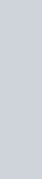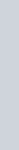BUFFER SOLUTIONSBUFFER

SOLUTIONS

A buffer solution is a solution that resists changes in its pH when an acid or a base is added to it.

Two types of mixtures form buffer solutions: a weak acid and a salt of the weak acid a weak base and a salt of the weak base

# BUFFERING ACTION

For a buffer containing a weak acid and a salt of the weak acid. weak acid: HA salt of weak acid: NaA (A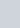This buffer solution contains a reaction at equilibrium.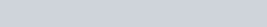This buffer solution contains significant amounts of both HA and A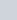What happens if H* is added (e.g., from HCI)? A- reacts with the added H*.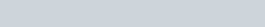This is buffering action. Most of the added 1-1+ is consumed, so the pH changes only slightly.

What happens if OH- is added (e.g., from NaOH)?

HA reacts with the added OH¯.

OH— + HA 1-120 +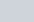This is buffering action. Most of the added OH¯ is consumed, so the pH changes only slightly.

For a buffer containing a weak base and a salt of the weak base. weak base: M salt ofweak base: (MH)CI (MH+)

This buffer solution contains a reaction at equilibrium.

## M + H20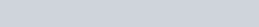This buffer solution contains significant amounts of both M and MH+.

What happens if H+ is added (e.g., from HCI)? M reacts with the added

This is buffering action. Most of the added is consumed, so the pH changes only slightly.

What happens if OH¯ is added (e.g., from NaOH)?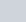reacts with the added OH¯.

##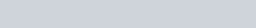H20 + M

This is buffering action. Most of the added OH- is consumed, so the pH changes only slightly.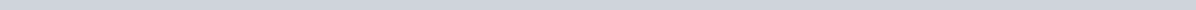A.B. Exercise OneA buffer solution contains HC2H302 and NaC2H302.Write the equation for the buffering action that occurs when HCI is added to this buffer solution.Write the equation for the buffering action that occurs when NaOH is added to this buffer solution.Another buffer solution contains NH3 and NH4Cl.Write the equation for the buffering action that occurs when HCI is added to this buffer solution.Write the equation for the buffering action that occurs when NaOH is added to this buffer solution.

# CALCULATING THE pH OF A BUFFER SOLUTION

Example 1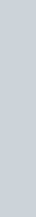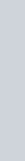Calculate the pH of a buffer solution prepared by dissolving 0.10 mole of cyanic acid, HCNO, and 0.50 mole of sodium cyanate, NaCNO, in enough water to make 0.500 liter of solution. For HCNO, = 2.0 x at 25 0C.

l. Write the equation for the equilibrium reaction and its K expression.

HCNO(aq) 4+(aq) + CNC(aq)[H+] [CNO-]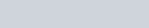## [HCNOI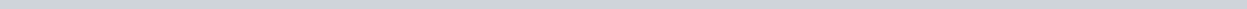1. Summarize the information in a table based on the chemical equation.

HCNO 1-1+ + CNO¯

 initial moles 0.100.5 initial conc 0.20 0 1equil

1. Test to see if the calculation can be simplified:

400 400 x (2.0 x 10-4) = 0.08

0.08 is less than both 0.20 and 1.0.

Therefore, assume 0.20 —x = 0.20 and 1.0 + x = 1.0.

1. Find the value ofx by substituting equilibrium concentrations into K expression.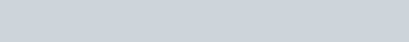– 2.0 x 104

 a [HCNOJ (0.20)0.20x (2.0 x 10-4 )1.0 x = 4.0 x 10 5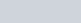= 4.0 x 10-5 M pH = -log(4.O x 10-5 ) = 4.40

 Exercise TwoA. Calculate the pH of a buffer solution prepared by dissolving 0.400 mole of formic acid, HCH02, and 0.100 mole of sodium formate, NaCH02, in 0.250 liter of solution. For formic acid, Ka = 1.77 x 104 at 25 0C.B. Calculate the pH of a buffer solution containing 0.20 mole ofNH3 and 0.15 mole of NH4Cl in 0.500 liter of solution. For NH3,Kb = 1.8 x 10-5 at 25 0C.

## CALCULATIONS INVOLVING BUFFERING ACTION

Adding a Base to a Weak Acid Buffer Solution

Example 2

Calculate the pH of the buffer solution in Example I after 0.020 mole ofNaOH has been added to it.

l . Write the equation for the equilibrium reaction and its K expression.

HCNO(aq) H+(aq) + CNO-(aq)

_ [H+] [CNO-] = 2.0k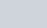### [HCNO]

1. Summarize the information in a table based on the chemical equation.

HCNO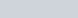H+ + CNOinitial conc 0.20 1.0

Now take into account the buffering action that results when 0.020 mole ofNaOH is added.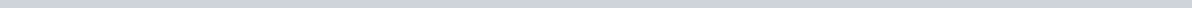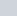HCNO CNO- + 1–120

0.020 mol 0.020 mol 0.020 mol added consumed formed

Therefore, as a result of buffering action,

0.020 molthe concentration of HCNO decreases by = 0.040 mol/L

0.500 L and

0.020 mol the concentration of CNO¯ increases by = 0.040 mol/L.

0.500 L

Put these results of buffering action into the table.

 HCNO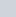CNO- initial conc 0.20 1.0 buff action —O.040 0 +0.040conc

change

equil

1. Simplifications can still be made.

Assume 0.16 —x = 0.16 and I .04 + x = 1.04.

1. Find the value ofx by substituting equilibrium concentrations into K expression.

_ [H+] [CNO-] =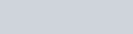= 2.0 x 104 [HCNO] (0.16)

0.16

x (2.0 x 10-4 )

1.04 x = 3.1 x 10 5

[W] = x = 3.1 x M

pH = -log(3.1 x 10-5 ) = 4 51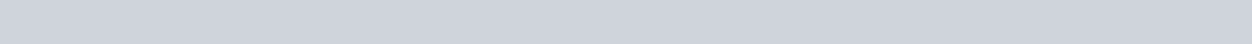Exercise ThreeA buffer solution contains 0.400 mole of formic acid, HCH02, and 0.100 mole of sodium formate, NaCH02, in I .00 liter of solution. Calculate the pH of this buffer solution after0.100 mole ofNaOH is added. For HCH02, 1.77 x at 25 0C.

## CALCULATIONS INVOLVING BUFFERING ACTION

Adding an Acid to a Weak Acid Buffer Solution

Example 3

Calculate the pH of the buffer solution from Example I after 0.030 mole of HCI is added.

Take into account the buffering action that results when 0.030 mole of HCI is added.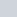CNO- HCNO

0.030 mol 0.030 mol 0.030 mol added consumed formed

Therefore, as a result of buffering action,

0.030 mol the concentration of CNO- decreases by = 0.060 mol/L

0.500 L and

0.030 mol the concentration of HCNO increases by = 0.060 mol/L.

0.500 L

Put these results of buffering action into the table.

HCNO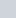CNO-

initial conc 0.20 1.0 buff action +0.060 -0.060conc

change

equil

Make simplifications:

Assume 0.26 —x = 0.26 and 0.94 + x = 0.94.

Find the value of x by substituting equilibrium concentrations into K expression.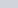[H+] [CNO-] (x) (0.94) = 2.0 x 10-4

[HCNO] (0.26)

0.26x (2.0 x 104 )

0.94

x = 5.5 x 10-5 pH = -log(5.5 x 10-5 ) = 4.26

 Exercise FourA buffer solution contains 0.400 mole of formic acid, HCH02, and 0.100 mole of sodium formate, NaCH02, in I .00 liter of solution. Calculate the pH of this solution after 0.050 mole of HCI is added. For HCH02, 1.77 x 10-4 at 25 0C.## CALCULATIONS INVOLVING BUFFERING ACTION

Buffering Action in a Weak Base Buffer ?olution

### Example 4

A buffer solution is prepared by dissolving 0.100 mole of methylamine, CH3NH2, and 0.0600 mole of methylammonium chloride, CE%NH3Cl, in 0.250 liter of solution. Calculate the pH of this solution after 0.0200 mole of HCI has been added. For CH3NH2, Kb = 4.38 x 10l . Write the equation for the equilibrium reaction and its K expression.

CH3NH2(aq) + H20(l) CH3NH3+(aq) + OH-(aq)

#### [CH3NH3+] [OH-I 4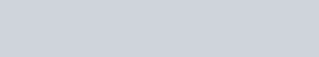= 4.38 x 10

2. Summarize the information in a table based on the chemical equation. The volume of the solution is 0.250 L.

#### CH3NH2 + 1-120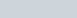CH3NH3+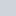initial moles 0.100 0.0600 initial conc 0.400 0.240

Take into account the buffering action. The added H+ is consumed by CH3NH#### + CH3NH2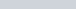CH3NH3+

0.020 mol 0.020 mol 0.020 mol added consumed formed

Therefore, as a result of buffering action,

0.0200 mol the concentration of CH3NH2 decreases by 0.250 L = 0.0800 mol/L

and

0.0200 mol the concentration of CH3NH3 increases by 0.250 L = 0.0800 mol/L.

#### CH3NH2 + H20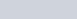CH3NH3+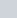initial conc 0.400 0.24 o buff action —0.0800 0.08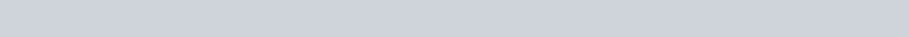at equil 0.320-x 0.320+x x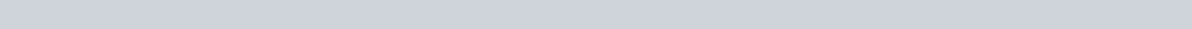1. Simplifications:

[CH3NH2] = 0.320-x=o.320 [CH3NH3+] = 0.320 + x = 0.320

[OH-I = x

1. Solve for x and find pH.

[CH3NH3+] [OH-J _ (0.32) (x)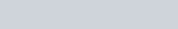[CH3NH2] (0.32)

0.32

x (4.38 x 104 )

0.32

x = 4.38 x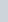= [OH-I

POH = -log(4.38 x 104) = 3.358

pH = 14.000- p0H= 14.000-3.358 = 10.642

 A. Exercise FiveA buffer solution solution. Calculate to it. For NH3, contains 0.20 mole NH3 and 0.60 mole NH4Cl in 750 mL of the pH of this buffer solution after 0.10 mole ofNaOH is added 1.8 x 10-5 at 250C.B. One liter of a buffer solution is prepared so that it contains 0.10 mole ofNH3 and 0.10 mole ofNH4Cl. When one mole ofNaOH is added to this solution, the pH changes drastically. Why did the buffer solution fail to maintain its pH?

## PROBLEMS

l . a. Calculate the pH of a solution made by adding 0.50 mole of nitrous acid, HN02, and

0.10 mole of potassium nitrite, KN02, to enough water to make 1.0 liter of solution. The Ka for HN02 is 4.5 x 10-4 at 25 0C.

b. Calculate the pH of this solution after 0.010 mole of HCI is added to it.

2. Ethyl amine, C2H5NH2, ionizes in aqueous solution according to the equation

C2H5NH2 + H20 C2H5NH3+ + OHThe value of Kb for this reaction is 5.6 x 104 at 25 0C.

1. Calculate the pH of a solution made by adding 0.20 mole of C2H5NH2 and 0.10 mole of ethylammonium chloride, (C2H5NH3)Cl, to enough water to make 0.50 liter of solution.
2. Calculate the pH of this solution after 0.010 mole of HCI has been added to it.

Reaction Order and Rate Law Expression Worksheet

Given the following equations and experimental data, write the correct

1. Rate Law Expression
2. Reaction Order
3. Determine k, the Specific Rate Constant (including units)

1.A2 + B2 2 AB

 Exp # [A2] [B2] Rate (mole dm-3 s-1) 1 0.001 0.001 0.01 2 0.001 0.002 0.02 3 0.001 0.003 0.03 4 0.001 0.004 0.04 5 0.002 0.004 0.16 6 0.003 0.004 0.36

a.

b.

c.

1.C + D E

 Exp # [C] [D] Rate (mole dm-3 s-1) 1 0.1 0.01 0.02 2 0.1 0.02 0.04 3 0.1 0.03 0.06 4 0.1 0.04 0.08 5 0.2 0.04 0.08 6 0.3 0.04 0.08

a.

b.

c.

#F + G H

 Exp # [F] [G] Rate (mole dm-3 s-1) 1 0.01 0.4 0.02 2 0.02 0.4 0.04 3 0.03 0.4 0.06 4 0.1 0.2 0.10 5 0.1 0.4 0.20 6 0.1 0.6 0.30

a.

b.

c.

#C + D E

 Exp # [C] [D] Rate (mole dm-3 s-1) 1 0.1 0.01 0.02 2 0.1 0.02 0.08 3 0.1 0.03 0.18 4 0.1 0.04 0.32 5 0.2 0.04 1.28 6 0.3 0.04 2.88

a.

b.

c.

#F + G H

 Exp # [F] [G] Rate (mole dm-3 s-1) 1 0.01 0.4 0.02 2 0.02 0.4 0.16 3 0.03 0.4 0.54 4 0.1 0.2 5 5 0.1 0.4 20 6 0.1 0.6 45

a.

b.

c.

Reaction Order and Rate Law Expression Worksheet

Given the following equations and experimental data, write the correct

1. Rate Law Expression
2. Reaction Order
3. Determine k, the Specific Rate Constant (including units)

1.A2 + B2 2 AB

 Exp # [A2] [B2] Rate (mole dm-3 s-1) 1 0.001 0.001 0.01 2 0.001 0.002 0.02 3 0.001 0.003 0.03 4 0.001 0.004 0.04 5 0.002 0.004 0.16 6 0.003 0.004 0.36

a.

b.

c.

1.C + D E

 Exp # [C] [D] Rate (mole dm-3 s-1) 1 0.1 0.01 0.02 2 0.1 0.02 0.04 3 0.1 0.03 0.06 4 0.1 0.04 0.08 5 0.2 0.04 0.08 6 0.3 0.04 0.08

a.

b.

c.

1.F + G H

 Exp # [F] [G] Rate (mole dm-3 s-1) 1 0.01 0.4 0.02 2 0.02 0.4 0.04 3 0.03 0.4 0.06 4 0.1 0.2 0.10 5 0.1 0.4 0.20 6 0.1 0.6 0.30

a.

b.

c.

1.C + D E

 Exp # [C] [D] Rate (mole dm-3 s-1) 1 0.1 0.01 0.02 2 0.1 0.02 0.08 3 0.1 0.03 0.18 4 0.1 0.04 0.32 5 0.2 0.04 1.28 6 0.3 0.04 2.88

a.

b.

c.

1.F + G H

 Exp # [F] [G] Rate (mole dm-3 s-1) 1 0.01 0.4 0.02 2 0.02 0.4 0.16 3 0.03 0.4 0.54 4 0.1 0.2 5 5 0.1 0.4 20 6 0.1 0.6 45

a.

b.

c.

CHAPTER 13- Practice Exercise Dr. PAHLAVAN

# Chemical Kinetics

Problem #1. Consider reaction; 2N2O (g) ® 2N2 (g) + O2 (g)

1. Write three different expressions which might be used to describe rates of reaction. (b) Relate the three rates to each other and explain the relative rate.

Problem #2. Consider the reaction; 2NO (g) + 2H2 (g) ® N2 (g) + 2H2O (g) (a) Write the rate law expression for reaction.

1. If rate law is = K[NO]2[H2],
1. determine the overall order and solve equation for rate constant.
2. Write unit of K if the unit of concentration is M or atm.
2. How does the initial rate change if the initial concentration of NO(g) is doubled and that of H2 (g) remains constant?
3. How does the initial rate change if the initial concentration of H2 (g) is doubled and that of NO(g) remains constant?

Problem #3. Use the table of data shown below to calculate the average rate of the reaction ; 2NO + Br2 ® 2NOBr

(a) between 10 sec and 30 sec (b) after 25 sec

Time (seconds): 0 10 20 30 40

[NO] mol/L : 0.124 0.110 0.088 0.073 0.054

Problem #4. The half-life of a first order reaction is 6.00×10-2 s. What is the rate constant for the reaction?

Problem #5. The rate constant for the first-order decomposition of ; 2N2O5 (g) ® 4NO2 (g) + O2 (g) at 25 oC is 6.32 x10-4 s-1.

1. What is the concentration of N2O5 remaining after 2.00 hrs, if initial concentration of N2O5 was 0.500M?
2. How long will it take (time required) for 90% of the N2O5 to decompose?
3. How long will it take for 90% to remain? (d) How long will it take for 50% to remain?

Problem #6. The following data were collected in three different experiments for the reaction; A + B ® C

EXP # [A]0, M [B]0, M initial rate, Ms-1

1. 0.20 1.0 5.0×10-2
2. 0.20 2.0 2.0×10-1
3. 0.40 2.0 4.0×10-1
1. Determine the rate law for the reaction as a function of the concentrations of A and B.
2. What is the overall order of the reaction? (c) Determine the rate constant (K) value.

(d) Determine the rate if [A]0 = 0.30 M and [B]0 = 0.10 M.

Problem #7. Given the following kinetic data for the reaction ; 2A + B + C ® 2D

[A]0, M [B]0, M [C]0, M initial rate, Ms-1

0.1 0.1 0.1 1.0

0.2 0.1 0.1 4.0

0.2 0.2 0.1 32.0

0.2 0.1 0.2 8.0

Determine the rate law as a function of concentration of A, B, and C, and determine the overall order of the reaction.

Problem # 8. The differential rate law for the association of iodine atoms to molecule iodine, 2I (g) ® I2 (g) is given rate = k[I]2 , where k = 7.0 x 109

a. What are the proper units for k? b. If [I]0 = 0.40 M, calculate[I] at t=2.5×10-7 sec. c. If [I]0 = 0.40 M, calculate t1/2.

d. If [I]0 = 0.80 M, how much time would it take for 75% of the iodine atoms to react?

Problem # 9. a) Consider the first order reaction 2N2O5(g) à 2N2O4 (g) + O2 (g) . The frequency factor A is 8.7×1012 s-1 and the activation energy is 63 kJ /mol. What is the rate constant for reaction at 75 oC?

b) Consider the decomposition of N2O5 from the following data: N2O4 (g) è 2NO2 , Ea = 108 kJ/mole

at T = 35o C k = 6.60×10-5 s-1. Calculate temperature when k = 2.40×10-3 s-1

Problem #10. Calculate the activation energy for the decomposition reaction of NO2 (g): 2NO2 (g) ® 2NO (g) + O2 (g) given that the rate constant at 300 oC is 5.7 x 10-1 M-1s-1 and at 230 oC it is 2.7 x 10-2 M-1s-1.

Problem # 11. An alteration in the structure of a certain virus follows first-order kinetics with an activation energy of 587 kJ/mol. The half-life of the reaction at 29.6 0C is 1.62×104s (1yr = 3.154 x 107s). What are the rate constant at 29.6 oC and the half-life at 32.0 oC for the alteration of structure of the virus?

Problem # 12. Consider the following elementary reactions (process)

1. CO (g) + Cl2 (g) ® COCl2 (g) II) HClI (g) ® HCl (g) + I (g)

What is the molecularity of each reaction and write the rate law expression for each? Problem # 13. Given the following mechanism for a reaction. I) Cl2 (g) ® 2Cl (g) ( slow)

1. 2NO (g) + 2Cl (g) ® N2 (g) + 2ClO (g) (fast) III) 2ClO (g) ® Cl2 (g) + O2 (g) (fast)

(a) Identify intermediate and catalyst (if any). (b) What is the molecularity of step(III)?

1. Which step determines the reaction rate and write rate law for that step.
2. Write the overall reaction equation and write rate law for overall reaction.

Problem #14. For the reaction; 2H2 (g) + 2NO (g) ® N2 (g) + 2H2O (g) ; show that the following proposed mechanism

is consistent with the experimental rate law: rate= [H2][NO]2

K1

1. 2NO (g) N2O2 (g) (fast)

K 1

K2

1. N2O2 (g) + H2 (g) H2O2 (g) + N2 (g) (slow, rate det.)

K3

1. H2 (g) + H2O2 (g) 2H2O (g) ( fast)

K 3

Problem #15. Describe the differences between the following and give examples;

a) catalyst and intermediate b) absorption and adsorbtion c) homogeneous and heterogeneous catalyst

d) first-order and second-order reaction e) average rate and instantaneous rate

Chemical Kinetics/Reaction Rate

Multiple Choice

1. Select the rate law that corresponds to the data shown for the following reaction:

 2 A + 3 B ® 2 C Experiment Initial [A] Initial[B] Initial Rate 1 0.15 0.12 0.10 2 0.15 0.24 0.20 3 0.45 0.24 1.80 a) R=k[A]3[B] b) R=k[A]3 2. Consider the following reaction:A ® 2 C c) R=k[A]2[B]2 d) R=k[A]2[B]

The average rate of appearance of C is given by W[C]/Wt. How is the average rate of appearance of C related to the average rate of disappearance of A?

a) – 2W[A]/Wt b) W[A]/Wt c) – W[A]/Wt d) – W[A]/2Wt

1. Which one of the following graphs shows the correct relationship between concentration and time for a second order reaction?
1. c)

log[A] 1/[A]

time time

1. d)

[A] [A]

time time

1. Which of the following will lower the activation energy for a reaction?
1. increasing the concentration of reactants
2. raising the temperature of the reaction
3. adding a suitable catalyst
4. all of the above

1. Consider the following reaction and its associated rate law:

A + B ® C R = k [A]2

Which of the following will not increase the rate of the reaction?

1. increasing the concentration of reactant A
2. increasing the concentration of reactant B
3. increasing the temperature of the reaction
4. adding a suitable catalyst

1. As the temperature of a reaction is increased, the rate of the reaction increases because the
1. reactant molecules collide less frequently
2. reactant molecules collide with greater energy
3. activation energy is lowered
4. reactant molecules collide less frequently and with greater energy

1. Which line in the reaction profile corresponds to the activation energy for the forward reaction?

Energy

Extent of Reaction

1. w b) x c) y d) z

1. In any multi-step reaction mechanism, the rate of the overall reaction is determined by the rate of the ___________ step in the mechanism.
1. first b) last c) slowest d) fastest

1. Consider the following hypothetical chemical reaction:

2 A + 2 B ® C The mechanism for this reaction is

1. A + B ® D (slow)
2. D + B ® E (fast)
3. A + E ® C (fast)

Which of the following is the correct rate law for this reaction?

1. R=k[A][B] b) R=k[A][E] c) R=k[A]2[B]2 d) R=k[D][B]

1. In the figure below, the quantity x is which of the five choices?

­ Potential

Energy

Reaction Coordinate ®

1. DE b) Ea (forward) c) Ea (reverse) d) the collision energy e) the steric factor

1. For a simple two-step elementary reaction:

Step 1 Step 2

Reactants ® Intermediates ® Products the potential energy profile is given below. Which one of the following statements applies? ­

Potential

Energy

Reaction Coordinate ®

1. The intermediates accumulate in measurable quantities, and the reaction is easily resolved into two steps
2. The reactants quickly form intermediates that slowly form products.
3. The presence of intermediates is difficult to determine kinetically although the two-step reaction is not ultra-fast.
4. The intermediates are the final products.
5. None of the above.

1. Which substance in the reaction below either appears or disappears the fastest?

4 NH3 + 7 O2 ® 4 NO2 + 6 H2O

1. NH3 b) O2 c) NO2 d) H2O

1. Which one of the following rate laws is for a reaction that is overall third order?
1. rate = k[NO]3[O2] c) rate = k[NH3]3[O2]3

[OsO2]3

1. rate = k[C6H8]2[H2]2 d) rate = k[H2O2]3

[H2]

1. Calculate the rate constant of a first order process that has a half-life of 225 s.
1. 0.693 s-1 b) 3.08 x 10-3 s-1 c) 1.25 s-1

1. What is the intermediate in the following mechanism?

A + B ® C + D B + D ® X

1. A b) B c) C d) D e) X

1. For the elementary reaction NO3 + CO ® NO2 + CO2
1. the molecularity is 2 and rate = k[NO3][CO]
2. the molecularity is 4 and rate = k[NO3][CO][NO2][CO2]
3. the molecularity is 2 and rate = k[NO2][CO2]
4. the molecularity is 2 and rate = k[NO3][CO]/[NO2][CO2]
5. the molecularity is 4 and rate = k[NO2][CO2]/[NO3][CO]

1. Given the following mechanism, which species below may be classified as intermediates in the formation of XO2 from X and O2?

X + YO2 ® XO + YO

XO + YO ® XO2 + YO

YO + O2 ® YO + O

YO + O ® YO2

a) X only b) YO only c) both X & YO2 d) both XO & YO

e) both YO2 & O2

1. Given the following mechanism, which of the species below is a catalyst in the formation of XO2 from X and O2?

X + YO2 ® XO + YO

XO + YO2 ® XO2 + YO

YO + O2 ® YO2 + O

YO O ® YO2

1. X b) O2 c) YO2 d) XO e) XO2

1. Chlorine atoms photochemically derived from Freons help destroy stratospheric ozone in the two-step mechanism below. We classify the respective species Cl and ClO for the overall balanced reaction as

Cl + O3 ® ClO + O2

ClO + O ® Cl + O2

1. both catalysts d) intermediate and catalyst
2. both intermediates e) both activated complexes

b) catalyst and intermediate

Problems (Show all work and include units)

1. Use the data table below to calculate the average rate of the reaction between 10 s and 30 s. A + B ® C

Time (seconds) [A] mol/L

0 0.124

10 0.110

20 0.088

30 0.073

40 0.054

1. Assume that the reaction described below is first order in reactants A and B. Calculate the value of the reaction rate constant using data for experiment 1.

A + 3 B ® 2 C

 Experiment Initial [A] Initial [B] Initial Rate 1 0.15 0.12 0.10 2 0.15 0.24 0.20 3 0.45 0.24 1.80

1. The reaction rate constant for a particular second order reaction is 0.47 L/mol s. If the initial concentration of reactant is 0.25 mol/L, how many seconds will it take for the concentration to decrease to 0.13 mol/L.

1. The activation energy for the reaction 2 NO2 (g) ® 2 NO (g) + O2 (g) is 114 kJ/mol. If k = 0.75 M-1 s-1 at 600.0 0C, what is the value of k at 500.0 0C?

1. The mechanism,

k1

Step1 NO2 + O3 ® NO3 + O2 (slow)

k2

Step 2 NO3 + NO2 ® N2O5 (fast)

Has been proposed for a reaction.

1. Write the equation for the overall reaction.
2. Write the rate laws for steps 1 and 2.
3. Write the rate law predicted by the mechanism for the overall reaction.
4. Which species is an intermediate?
5. Determine the molecularity of step 1.

1. The reaction A ® B + C is carried out at a particular temperature. The observed data is recorded below. Graph the data to determine the order of the reaction, write the rate law for the reaction, and determine the value of k at this temperature.
 Time (min) [A] mol/L 0.00 2.000 2.00 1.107 4.00 0.612 6.00 0.338 8.00 0.187 10.00 0.103

1. For a certain reaction, the rate constant doubles when the temperature increases from 15.00C

To 25.00C. Calculate: a) the activation energy

b) the rate constant at 100.00C, taking k at 25.00C to be

1.2 x 10-2L/mol s

1. The decomposition of 2NOCl → 2NO(g) + Cl2(g) has k = 9.3×10-5 L/mol•s at 100˚C and k = 1.0×10-3 L/mol•s at 130˚C.
1. What is the activation energy for the reaction?
2. What is the value of k at 200°C?
2. Use the following data to determine the activation energy for the decomposition of HI:

Temperature (K) Rate Constant (M/s)

573 2.91 x 10-6

673 8.38 x 10-4

773 7.65 x 10-2

1. The rate constant for the reaction H2(g) + I2(g) —> 2HI(g) is 5.4 x 10-4 M-1s-1 at 326 oC. At 410 oC the rate constant was found to be 2.8 x 10-2 M-1s-1.

Calculate the a) activation energy and b) rate constant for this reaction at 6300C.

1. If a reaction’s rate constant at 298K is 33 M-1s-1 and 45 M-1s-1 at 675 K, what is the activation energy?
2. Suppose the activation energy of a certain reaction is 250 kJ/mol. If the rate constant at T1 = 300 K is k1 and the rate constant at T2 = 320 K is k2, then the reaction is __ times faster at 320 K than at 300 K. (Hint: Solve for k2/k1.)

(a) 3 x 1029 (b) 0.067 (c) 15.0 (d) 525 (e) 3 x 1028

1. What is the activation energy (in kJ) of a reaction whose rate constant increases by a factor of 100 upon increasing the temperature from 300 K to 360 K? (a) 27 (b) 35 (c) 42 (d) 53 (e) 69
2. A second-order reaction was observed. The reaction rate constant at three degrees Celsius was found to be 8.9 x 10-3 L/mol and 7.1 x 10-2 L/mol at 35 degrees Celsius. What is the activation energy of this reaction?
3. Changing the temperature of a reaction from 273K to 300 K causes the rate constant, k, to increase by a factor of seven. Calculate Ea for this reaction (R=8.314 J/mol K)
4. The uncoiling of DNA is a 1st order process with an activation energy of about 420 kJ/mol. The half-time at 50o C is about 2 minutes. What is the half-time at normal body temperature, 37oC.
5. Use the following information to determine the activation energy for the reaction shown here:

A + B → C + D

Temperature (K) Rate Constant (L/mol·s)

312 0.0339

372 0.119

1. The rate constant for the following reaction was determined at two different temperatures: BH4(aq) + NH4+(aq) → BH3NH3(aq) + H2(g) The data is shown in the table below.

 T (oC) k (M-1s-1) T (K) 1/T (K-1) lnk (n unit) 30.0 1.94×10-4 303 3.30003 x 10-3 -8.5477 40.0 1.49×10-3 313 3.19489 x 10-3 -6.5090

1. Calculate the activation energy for the reaction.

1. Calculate the rate constant for the reaction at 100°C.

1. The rate constant of a first order reaction is 3.46×10-2 s-1 at 298K. What is the rate constant at 350K if the activation energy for the reaction is 50.2kJ/mol?

1. A reaction is known to have an activation energy of 70.0kJ/mol. If the rate constant at 148°C is

21.0 M-1 s-1, what will the rate constant be at 246°C?

## Integrated Rate Law and Half Life Sample Problems

1. The rate of the reaction O(g) + NO2(g) à NO(g) + O2(g) was studied at a certain temperature.
1. In the first set of experiments, NO2 was in large excess, at a concentration of 1.0 x 1013 molecules/cm3 with the following data collected:
 Time (s) [O] (atoms/cm3) 0 5.0 x 109 1.0 x 10-2 1.9 x 109 2.0 x 10-2 6.8 x 108 3.0 x 10-2 2.5 x 108

What is the order of the reaction with respect to oxygen atoms?

1. The reaction is known to be first order with respect to NO2. Determine the overall rate law and the value of the rate constant.

1. The decomposition of a certain insecticide in water follows first-order kinetics with a rate constant of 1.45 yr-1. A quantity of this insecticide is washed into a lake on June 1, leading to a concentration of 5.0 x 10-7 g/cm3.
1. What is the concentration of the insecticide on June 1 of the following year?

1. How long will it take for the concentration of the insecticide to decrease to 3.0 x 10-7 g/cm3?

1. The decomposition of dimethyl ether, (CH3)2O at 510º C is a first-order process with a rate constant of 6.8 x 10-4 s-1.

(CH3)2O(g) à CH4(g) + H2(g) + CO(g)

If the initial pressure of (CH3)2O is 135 torr, what is its pressure after 1420 s?

1. Consider again the decomposition of NO2 discussed in slides 39-41. The reaction is second order in NO2 with k = 0.543 1/Ms. If the initial concentration of NO2 in a closed vessel is 0.0500 M, what is the remaining concentration after 0.500 hr?

1. Calculate t1/2 for the decomposition of the insecticide described on slide 35. How long does it take for the concentration of the insecticide to reach one-quarter of the initial value?

1. The reaction of C4H9Cl with water is a first order reaction. Slide 12 shows how the concentration of C4H9Cl changes with time at a particular temperature. (a) From that graph, estimate the half-life for this reaction. (b) Use the half-life from (a) to calculate the rate constant.

NUCLEAR CHEMISTRY

BALANCING NUCLEAR REACTIONS WORKSHEET

Predict the missing product or reactant in the following nuclear reactions. Determine the type of nuclear reaction (α emission, β emission, γ emission, positron emission, artificial transmutation, fission, or fusion) described.

Type of Nuclear Reaction

1. 4219 K  0-1 e + _____ 1.)
2. 23994 Pu  42 He + _____ 2.)
3. 23592 U  _____ + 23190 Th 3.)
4. 11 H + 31 H  _____ 4.)
5. 63 Li + 10 n  42 He + _____ 5.)
6. 2713 Al + 42 He  3015 P + _____ 6.)
7. 94 Be + 11 H  _____ + 42 He 7.)
8. 3719 K  0+1 e + _____ 8.)
9. _____ + 10 n  14256 Ba + 9136 Kr + 3 10 n 9.)
10. 23892 U + 42 He  _____ + 10 n 10.)
11. 146 C  147 N + _____ 11.)
12. 18775 Re + _____  18875 Re + 11 H 12.)
13. 2211 Na + _____  2210 Ne 13.) —
14. 21884 Po  _____ + 42 He 14.)
15. 25399 Es + 42 He  10 n + _____ 15.) Write the Balanced nuclear equations for the alpha decay of: a) Plutonium-234

b) Strontium-90

Write the balanced nuclear equations for the alpha, beta and gamma decay of Radium-226

1

Worksheet: pH Calculations Name______________

The letters “pH” represent the French words “pouvoir hydrogene” which means “hydrogen power”.

• The definition of pH is pH is equal to the negative log (logarithm) of the ____________ ion concentration of a solution.
• The logarithm of a number is the power to which 10 must be raised to equal that number.

A pH value of less than 7 indicates a(n) _______________ solution. A pH value of _____ indicates a neutral solution. A pH value of more than 7 indicates a(n) _______________ solution.

PROBLEMS: Show all work and circle the final answer.

1. Determine the pH of a 0.010 M HNO3 solution.

1. What is the pH of a 2.5 x 10-6 M solution of HCl?

1. Calculate the pH of a solution of 0.0025M H2SO4.

1. Calculate the pH of a 0.0010 M NaOH solution.

1. What is the pH of a 0.020M Sr(OH)2 solution?

CHEMISTRY: A Study of Matter

11.10

1. a) What is the hydrogen ion concentration of an aqueous HCl solution that has a pH of 3.0?

1. What is the hydroxide ion concentration of this same solution?

1. Which ion, H+ or OH, is in greater concentration? ____________

1. Is this solution acidic or basic? _____________

1. Find the [H+] and the [OH] of a solution with a pH of 3.494.

Is this solution acidic or basic?_____________________

CHEMISTRY: A Study of Matter

11.11

Weak Acids and Bases worksheet Name ____________________________

1. Find the pH of a 0.325 M acetic acid solution. Ka = 1.8 x 10-5.

1. Find the pH of a 0.056 M propionic acid solution (Ka = 1.4 x 10-5).

1. Find the pH of a 0.065 M solution of formic acid. The acid dissociation constant (Ka) for formic acid is 1.8 x 10-4.

1. Find the pH of a 0.15 M solution of ammonia, NH3. Kb = 1.8 X 10-5

1. Find the pH of a 0.600 M solution of methylamine CH3NH2. Kb = 4.4 x 10–4.Equilibrium Worksheet

1. 2NH3(g)  N2(g) + 3H2(g)

At 500 K, the following concentrations were measured: [N2] = 3.0 x 10-2 M, [H2] = 3.7 x 10-2 M, [NH3] = 1.6 x 10-2 M. What is Kc?

1. At 1000 K, the equilibrium partial pressures for the reaction below are: CH4 = 0.20 atm, H2S = 0.25 atm, CS2 = 0.52 atm, and H2 = 0.10 atm. What is Kp? CH4(g) + 2H2S(g)  CS2(g) + 4H2(g)

1. N2(g) + 3H2(g)  2 NH3(g) At 375 C, Kc = 2.79×10-5.
1. What is Kp?

1. What is PNH3 if PH2 = 1.24 atm and PN2 = 2.17 atm at equilibrium?

1. Given the equations:
 H2(g) + S(s)  H2S(g) Kc = 1.0×10-3 S(s) + O2(g)  SO2(g) Kc = 5.0×106

Calculate the value of Kc for H2(g) + SO2(g)  H2S(g) + O2(g)

1. For the reaction, B  2A, Kc = 2. Suppose 3.0 moles of A and 3.0 moles of B are introduced into a 2.00 L flask.

[A] = [B] = Q =

1. Is this system at equilibrium?
2. In which direction will the reaction proceed to reach equilibrium?
3. As the system moves towards equilibrium, what happens to the concentration of B? A?

1

1. a) Calculate the equilibrium concentrations of all species for the following reaction if the initial concentrations of H2 and I2 are both 1.00 M. H2 (g) + I2 (g)  2 HI (g) Kc = 50.5

b) For the reaction above, if Kp = 50.5 and the initial pressures are HI = 0.975 atm, H2 = 0.105 atm and I2 = 0.105 atm, what are the equilibrium pressures for all the substances?

7. Calculate the equilibrium concentrations for the reaction below if the initial [N2] = 0.80 M and the initial [O2] = .20 M

N2(g) + O2(g)  2 NO(g) Kc = 1.0 x 10-5

6. Calculate the equilibrium concentrations of all species if 3.000 moles of H2 and 6.000 moles of F2 are placed in a 3.000 L container.

H2(g) + F2(g)  2HF(g), Kc = 1.15 x 102

2

Ksp and Molar Solubility Problems Worksheet

1. Use the chemical AgCl to describe solubility, molar solubility and solubility product

1. Write balanced equations and solubility product expressions for the following compounds
1. CuBr d. Hg2Cl2

1. ZnC2O4 e. AuCl3

1. Ag2CrO4 f. Mn3(PO4)3

1. Silver Chloride has a larger Ksp than silver carbonate (Ksp = 1.6×10‐10 and 8.1×10‐12 respectively). Does this mean that AgCl also has a larger molar solubility than Ag2CO3? Explain.

1. Calculate the concentration of ions in the following saturated solutions
1. [I] in AgI solutions with [Ag+] = 9.1×10‐9
2. [Al3+] in Al(OH)3 solution with [OH] = 2.9 x10‐9

1. From the solubility data given, calculate the solubility product for the following compounds: a. SrF2 7.3×10‐2 g/L

b. Ag3PO4 6.7×10‐3 g/L

1. The molar solubility of MnCO3 is 4.2×10‐6 M. What is Ksp for this compound?

1. If 20.0 mL of 0.10 M Ba(NO3)2 are added to 50.0 mL of 0.10 M Na2CO3, will BaCO3 precipitate? Supply explanation / calculations to support answer.

1. A volume of 75 mL of 0.060 M NaF is mixed with 25 mL of 0.15 M Sr(NO3)2. Calculate the concentrations in the final solution of NO3, Na+, Sr2+, and F. (Ksp for SrF2 = 20.x10‐10)

 9. Calculate the Ksp for each of the salts whose solubility is listed below. a.    b. CaSO4 = 5.0 x 10‐3 mol/LMgF2 = 2.7 x 10‐3 mol/L c.    d. AgC2H3O2 = 1.02 g/100 mLSrF2 = 12.2 mg/100 mL

1. Calculate
1. the solubility in moles/L of each of three salts and
2. the concentration of the cations in mg/mL in each of the saturated solutions. i. AgCN Ksp = 2.0 x 10‐12 ii. BaSO4 Ksp = 1.5 x 10‐9

iii. FeS Ksp = 3.7 x 10‐19 iv. Mg(OH)2 Ksp = 9.0 x 10‐12

1. Ag2S Ksp = 1.6 x 10‐49
2. CaF2 Ksp = 4.9 x 10‐11

1. Consider these slightly soluble salts:
1. PbS Ksp = 8.4 x 10‐28
2. PbSO4 Ksp = 1.8 x 10‐8
3. Pb(IO3)2 Ksp = 2.6 x 10‐13
1. Which is the most soluble?
2. Calculate the solubility in moles/L for PbSO4.
3. How many grams of PbSO4 dissolve in 1 L of solution? iv. How can you decrease the concentration of Pb2+(aq) in a saturated solution of PbSO4 solution?
4. What is the concentration in moles/L of PbS in a saturated solution of the salt?

1. For each of these substances, calculate the milligrams of metallic ion that can remain at equilibrium in a solution having a [OH] = 1.0 x 10‐4 mol/L.
1. Cu(OH)2 Ksp = 1.6 x 10‐9
2. Fe(OH)3 Ksp = 6.0 x 10‐38
3. Mg(OH)2 Ksp = 6.0 x 10‐12

1. The decomposition of 2NOCl → 2NO(g) + Cl2(g) has k = 9.3×10-5 L/mol•s at 100˚C and k = 1.0×10-3 L/mol•s at 130˚C.

a. What is the activation energy for the reaction?

b. What is the value of k at 200°C?

2. Use the following data to determine the activation energy for the decomposition of HI:

Temperature (K)           Rate Constant (M/s)

573                                  2.91 x 10-6

673                                  8.38 x 10-4

773                                  7.65 x 10-2

3. The rate constant for the reaction H2(g)  + I2(g) —> 2HI(g) is 5.4 x 10-4 M-1s-1 at 326 oC.     At 410 oC the rate constant was found to be 2.8 x 10-2 M-1s-1.

Calculate the a) activation energy and b) rate constant for this reaction at 6300C.

1. If a reaction’s rate constant at 298K is 33 M-1s-1 and 45 M-1s-1 at 675 K, what is the activation energy?
2. Suppose the activation energy of a certain reaction is 250 kJ/mol. If the rate constant at T1 = 300 K is k1 and the rate constant at T2 = 320 K is k2, then the reaction is __ times faster at 320 K than at 300 K. (Hint: Solve for k2/k1.)

(a) 3 x 1029 (b) 0.067 (c) 15.0 (d) 525 (e) 3 x 1028

1. What is the activation energy (in kJ) of a reaction whose rate constant increases by a factor of 100 upon increasing the temperature from 300 K to 360 K?

(a) 27 (b) 35 (c) 42 (d) 53 (e) 69

1. A second-order reaction was observed. The reaction rate constant at three degrees Celsius was found to be 8.9 x 10-3 L/mol and 7.1 x 10-2 L/mol at 35 degrees Celsius. What is the activation energy of this reaction?
2. Changing the temperature of a reaction from 273K to 300 K causes the rate constant, k, to increase by a factor of seven. Calculate Ea for this reaction (R=8.314 J/mol K)
3. The uncoiling of DNA is a 1st order process with an activation energy of about 420 kJ/mol. The half-time at 50o C is about 2 minutes. What is the half-time at normal body temperature, 37oC.
4.  Use the following information to determine the activation energy for the reaction  shown here:      A + B →  C + D

Temperature (K) Rate Constant (L/mol∙s)

312 0.0339

1. 0.119
2. The rate constant for the following reaction was determined at two different temperatures: BH4(aq) + NH4+(aq) → BH3NH3(aq) + H2(g) The data is shown in the table below.
 T (oC) k (M-1s-1) T (K) 1/T (K-1) lnk (n unit) 30.0 1.94×10‑4 303 3.30003 x 10-3 -8.5477 40.0 1.49×10‑3 313 3.19489 x 10-3 -6.5090
1. Calculate the activation energy for the reaction.
2. Calculate the rate constant for the reaction at 100°C.
3. The rate constant of a first order reaction is 3.46×10-2 s-1 at 298K. What is the rate constant at 350K if the activation energy for the reaction is 50.2kJ/mol?
4. A reaction is known to have an activation energy of 70.0kJ/mol. If the rate constant at 148°C is 21.0 M-1 s-1, what will the rate constant be at 246°C?